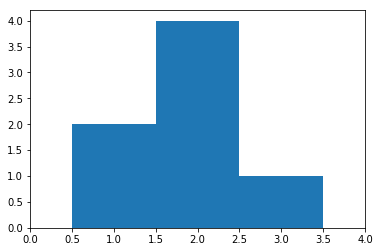# sciPy stats.histogram() function | Python

• Last Updated : 13 Feb, 2019

`scipy.stats.histogram(a, numbins, defaultreallimits, weights, printextras)` works to segregate the range into several bins and then returns the number of instances in each bin. This function is used to build the histogram.

Parameters :
arr : [array_like] input array.
numbins : [int] number of bins to use for the histogram. [Default = 10]
defaultlimits : (lower, upper) range of the histogram.
weights : [array_like] weights for each array element.
printextras : [array_like] to print the no, if extra points to the standard output, if true

Results :
– cumulative frequency binned values
– width of each bin
– lower real limit
– extra points.

Code #1:

 `# building the histogram ``import` `scipy``import` `numpy as np ``import` `matplotlib.pyplot as plt`` ` `hist, bin_edges ``=` `scipy.histogram([``1``, ``1``, ``2``, ``2``, ``2``, ``2``, ``3``],``                                       ``bins ``=` `range``(``5``))`` ` `# Checking the results``print` `(``"No. of points in each bin : "``, hist)``print` `(``"Size of the bins          : "``, bin_edges)`` ` `# plotting the histogram``plt.bar(bin_edges[:``-``1``], hist, width ``=` `1``)``plt.xlim(``min``(bin_edges), ``max``(bin_edges))``plt.show()`

Output :

```No. of points in each bin :  [0 2 4 1]
Size of the bins          :  [0 1 2 3 4]
```My Personal Notes arrow_drop_up